This assignment focuses on graphs, specifically on searching for paths in a graph. You will write several path finding algorithms.

We provide you with several support files, but you should not modify them. Turn in the following files;

1. trailblazer.cpp , code to perform graph path searches
2. map-custom.txt, a world map of your own creation.
3. map-custon.jpg, a world map of your own creation.

This is a pair assignment. You may work in a pair or alone. Find a partner in section or use the course message board. If you work as a pair, comment both members' names atop every code file. Only one of you should submit the program; do not turn in two copies. Submit using the Paperless system linked on the class web site.

Due Date: Trailblazer is due Dec 9th at 12:00pm.
Y.E.A.H hours:
Dec 2nd
3:30-4:30pm
Bishop Aud.

## Overview

This program displays various road maps and allows users to find the shortest path between any two nodes. For example, if the user loaded the Stanford map and selected a node at the top of the Oval and another node at FroSoCo, your program should display the best route: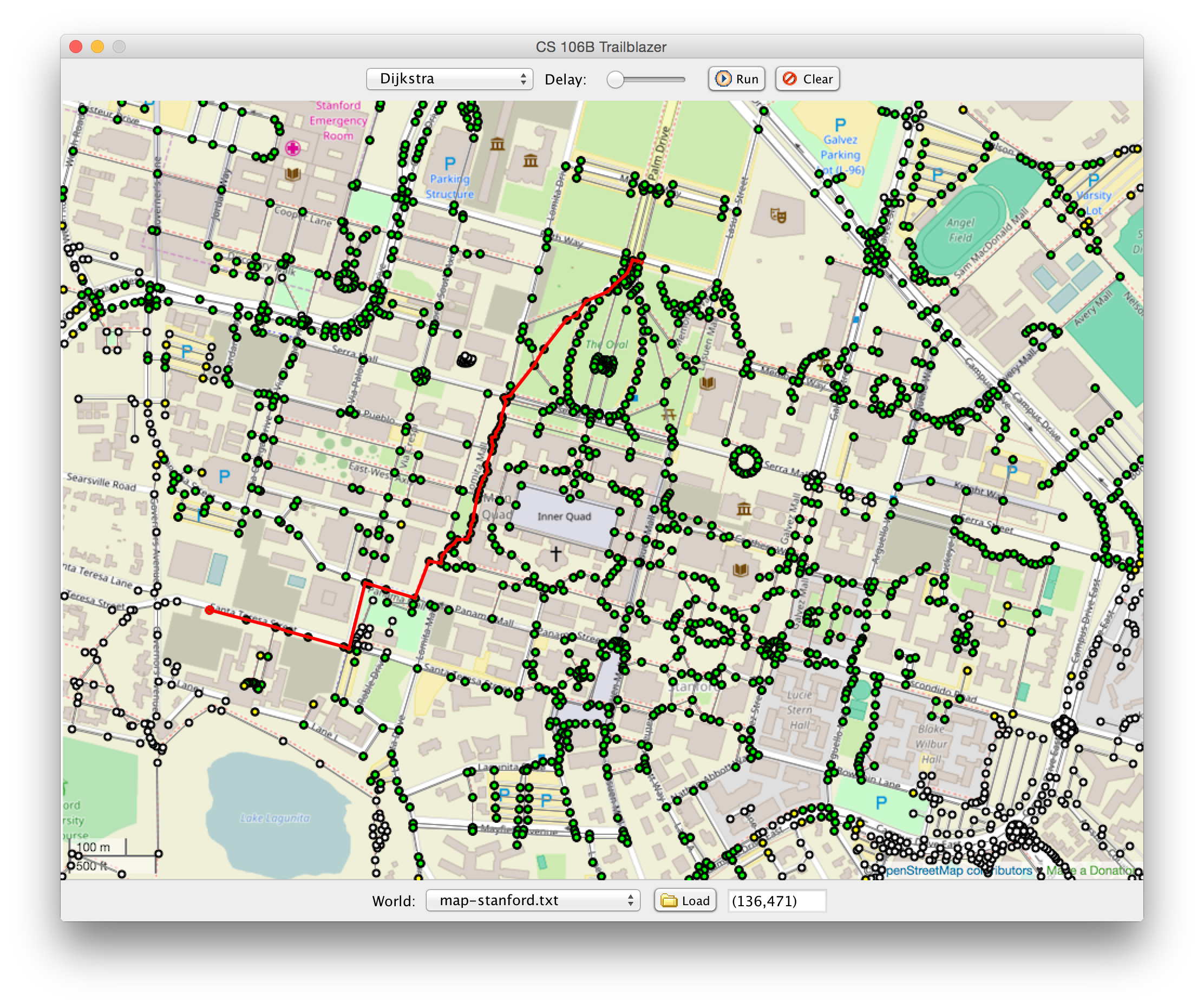If you click on any two nodes in the world, the program will find a path from the starting position to the ending position. As it does so, it will color the vertexes green and yellow based on the colors assigned to them by the algorithm. Once the path is found, the program will highlight it and display information about the path cost in the console. The user can select one of four path-searching algorithms in the top menu:

1. Breadth-first search (BFS)
2. Dijkstra's algorithm
3. A* search
4. Alternative Route

The window contains several controls. You can load world maps by selecting them from the bottom drop-down menu and then clicking the "Load" button.

In your trailblazer.cpp file, you must write the following 4 functions for finding paths and creating mazes in a graph:

Path breadthFirstSearch(RoadGraph& graph, Vertex* start, Vertex* end)
Path dijkstrasAlgorithm(RoadGraph& graph, Vertex* start, Vertex* end)
Path aStar(RoadGraph& graph, Vertex* start, Vertex* end)
Path alternativeRoute(RoadGraph& graph, Vertex* start, Vertex* end)

Each of the first three implements a path-searching algorithm taught in class. You should search the given graph for a path from the given start vertex to the given end vertex. If you find such a path, the path you return should be a list of all vertexes along that path, with the starting vertex first (index 0 of the vector) and the ending vertex last.

A Path is a typedef of a Vector<Vertex *>. It is an alias for the same exact type.

If no path is found, return an empty path. If the start and end vertexes are the same, return a one-element vector containing only that vertex. Though some graphs may be undirected (all edges go both ways), your code should not assume this. You may assume that the graph passed in is not corrupt.

Our provided main client program will allow you to test each algorithm one at a time before moving on to the next. You can add more functions as helpers if you like, particularly to remove redundancy between some algorithms containing similar code.

The road network world is represented by a RoadGraph, which is a thin wrapper around the BasicGraph we saw in class. Each vertex represents a specific location on the world. An edge between two verticies means that there is a direct road between the two. The cost of the path is the time it takes to traverse that road.

Your algorithm should work on any RoadGraph instance, such as this map of Middle Earth that can help Frodo get from the Shire to Mount Doom: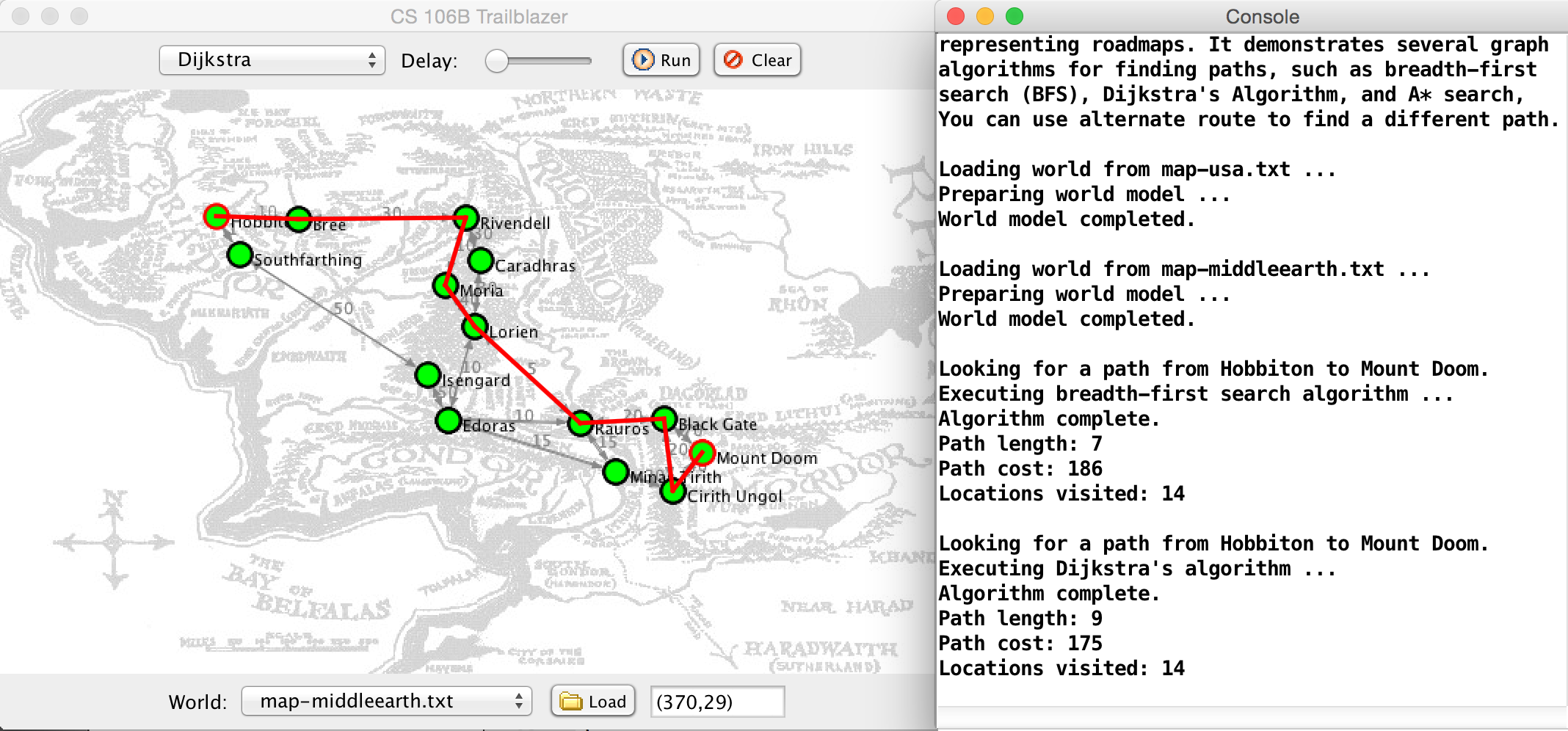## Provided Code

We provide you with a lot of starter code for this assignment. Here is a quick breakdown of what each file contains, though you do not need to examine or know about each file or its contents in order to complete the assignment.

1. trailblazer.h/.cpp: We provide a skeleton version of these files where you will write your path-searching code for the assignment.
2. Color.h/.cpp Constants representing colors of verticies
3. TrailblazerGUI.h/.cpp The app's graphical user interface.
4. trailblazermain/.cpp The main function that initializes the GUI and launches the application.
5. World(Abstract,Map).h/.cpp Hierarchy of types of world graphs.

## Vertex

Each vertex in the graph is represented by an instance of the Vertex structure, which has the following members:

Vertex Member Description
string name vertex's name, such as "r34c25" or "vertex17"
Set<Edge*> edges edges outbound from this vertex
void setColor(Color c) sets this vertex to be drawn in the given color in the GUI, one of UNCOLORED, WHITE, GRAY, YELLOW, or GREEN
string toString() returns a printable string representation of the vertex for debugging

When you call setColor on a Vertex, the GUI is automatically updated, the programs internal count of the number of verticies that you touch is increased, and a delay is added (so you can see your algorithm animate). All vertex colors are reset before each of your path-searching algorithms is called.

## Edge

Each edge in the graph is represented by an instance of the Edge structure, which has the following members:

Edge Member Description
Vertex* start the starting vertex of this edge
Vertex* finish the ending vertex of this edge (i.e., finish is a neighbor of start)
double cost cost to traverse this edge
string toString() returns a printable string representation of the vertex for debugging

## RoadGraph

The vertexes and edges are contained inside a RoadGraph object passed to each of your algorithm functions.

RoadGraph Methods Description
Set<Vertex *> getNeighbors(Vertex * v) get all Verticies that can be reached by a single edge from v
Edge * getEdge(Vertex * start, Vertex * end) return the edge that connects start to end
double getMaxRoadSpeed() the fastest speed of any edge in the graph
double getCrowFlyDistance(Vertex * start, Vertex * end) the distance between two nodes as the crow flies.

## Graph Algorithm Details

Coloring: In addition to searching for a path in each algorithm, we also want you to add some code to give colors to various vertexes at various times. This coloring information is used by the GUI to show the progress of your algorithm and to provide the appearance of animation. To give a color to a vertex, call the color member function on that vertex's Vertex object, passing it a global color constant such as GRAY, YELLOW, or GREEN. For example:

// set the start vertex's color to green
start->setColor(GREEN);

Here is a listing of colors available and when you should use them:

• enqueued = yellow: Whenever you enqueue a vertex to be visited for the first time, such as in BFS and Dijkstra's algorithm when you add a vertex to a data structure for later processing, color it yellow (YELLOW).
• visited = green: Whenever your algorithm directly visits and examines a particular vertex, such as when it is dequeued from the processing queue in BFS, color it green (GREEN).

The provided GUI has an animation slider that you can drag to set a delay between coloring calls. If the slider is not all the way to its left edge, each call to setColor on a vertex will pause the GUI briefly, causing the appearance of animation so that you can watch your algorithms run.

## Path Finding

See the expected output files for reference on what your program should do. For Dijkstra's and A* you should get the same path costs as shown in the expected outputs. But you do not need to exactly match our path itself, nor its "locations visited", so long as your path is a correct one

Check out the search handout for an example of the search algorithms. Each of the three search algorithms (BFS, Dijkstra, A*) follow the general paradigm: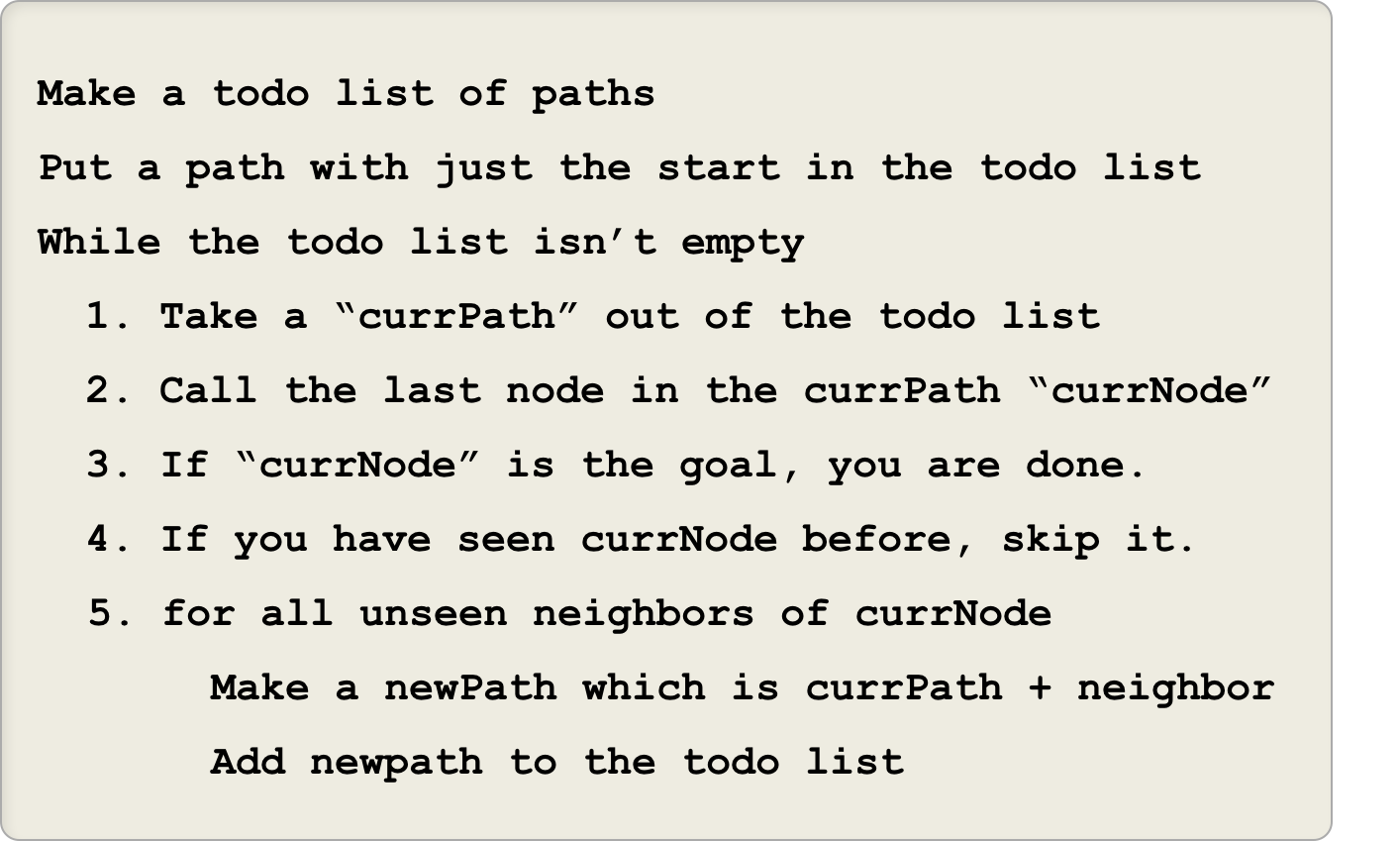Breadth-first search implementation notes: Implement the version of the algorithm pseudo-code from lecture. Make sure to keep track of nodes that you have already visited.

Dijkstra's algorithm implementation notes: The version of Dijkstra that we talk about in class is slightly different than the version in the book. Your implementation of Dijkstra's algorithm can follow any version. The one we talked about in lecture is probably the easiest. The priority queue should store paths, and once you find a path that ends in the destination, you should reconstruct the shortest path back. See the lecture slides for more details.

A* implementation notes: As discussed in class, the A* search algorithm is essentially a variation of Dijkstra's algorithm that uses heuristics to fine-tune the order of elements in its priority queue to explore more likely desirable elements first. So when you are implementing A*, you need a heuristic function to incorporate into the algorithm.

You can compare the behavior of Dijkstra's algorithm and A* (or any pair of algorithms). First try performing a search between two points using Dijkstra's algorithm, then select A* and press the "Run" button at the top of the GUI window. This will repeat the same search using the currently selected algorithm. Run a search using Dijkstra's algorithm, switch the algorithm choice to "A*," then run that search to see how much more efficient A* is.

## A* Heuristic

In order for A* to work, you need to define a heuristic function which under-estimates the cost of travelling between two vertices.

For a road map (where cost is travel time), a simple heuristic is to calculate the time it would take to get between the nodes assuming that there was a direct super highway between the two nodes. If the speed of a super highway is as fast or faster than the speed on any road then the algorithm will underestimate the true graph distance (which makes it an admissable heuristic). To calculate how long it would take to travel along a super highway between two nodes use the two RoadGraph functions: getCrowFlyDistance and getMaxRoadSpeed.

Recall that traveTime = distance / speed.

Your A* search algorithm should always return a path with the same length and cost as the path found by Dijkstra's algorithm. If you find that the algorithms give paths of different costs, it probably indicates a bug in your solution.

## Alternative Route

When travelling between two points on a road map, you may want to take the fastest route, but if there is a reasonable alternative you might prefer that instead. For example, though highway 101 is often slightly faster way to get to San Francisco, highway 280 is more beautiful (Chris Gregg thinks that you should also consider going all the way to highway 1). Your final task is to implement the alternativeRoute function.

In this example the shortest path between the oval and the back of MemChu is shown in red, and a next best alternative is shown in blue: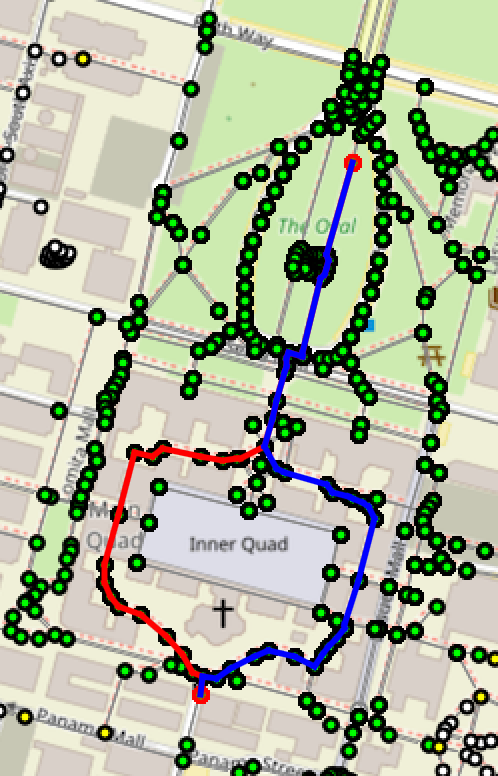We already know how to find a shorest path from a start to a goal. How do we find an alternate route?

To find an alternate path from a start node to an end node, first we are going to calculate the best (aka shortet) path between start and end. Then, for each edge in the best path we are going to calculate the shortest path from start to end that ignores that edge. Each search will return a candidate "alternate route". Your function should return the lowest cost alternate route that is sufficiently different from the original best path.

The difference of an alternate path P from the best path B is defined to be the number of nodes that are in B, but are not in P divided by the number of nodes in P:

$\text{difference}(P, B) = \frac{\text{# nodes in B that are not in P}}{\text{# nodes in B}}$

For each edge in the best path you will produce one candidate alternate route. Your function should choose, from those candidates, the shortest path that has a difference score greater than 0.2 when compared to the the original best path (see the constant SUFFICIENT_DIFFERENCE).

Example of alternative route calculation: In the graph bellow the shortest path from Start to End has four edges. For each edge we compute a candidate alternate route: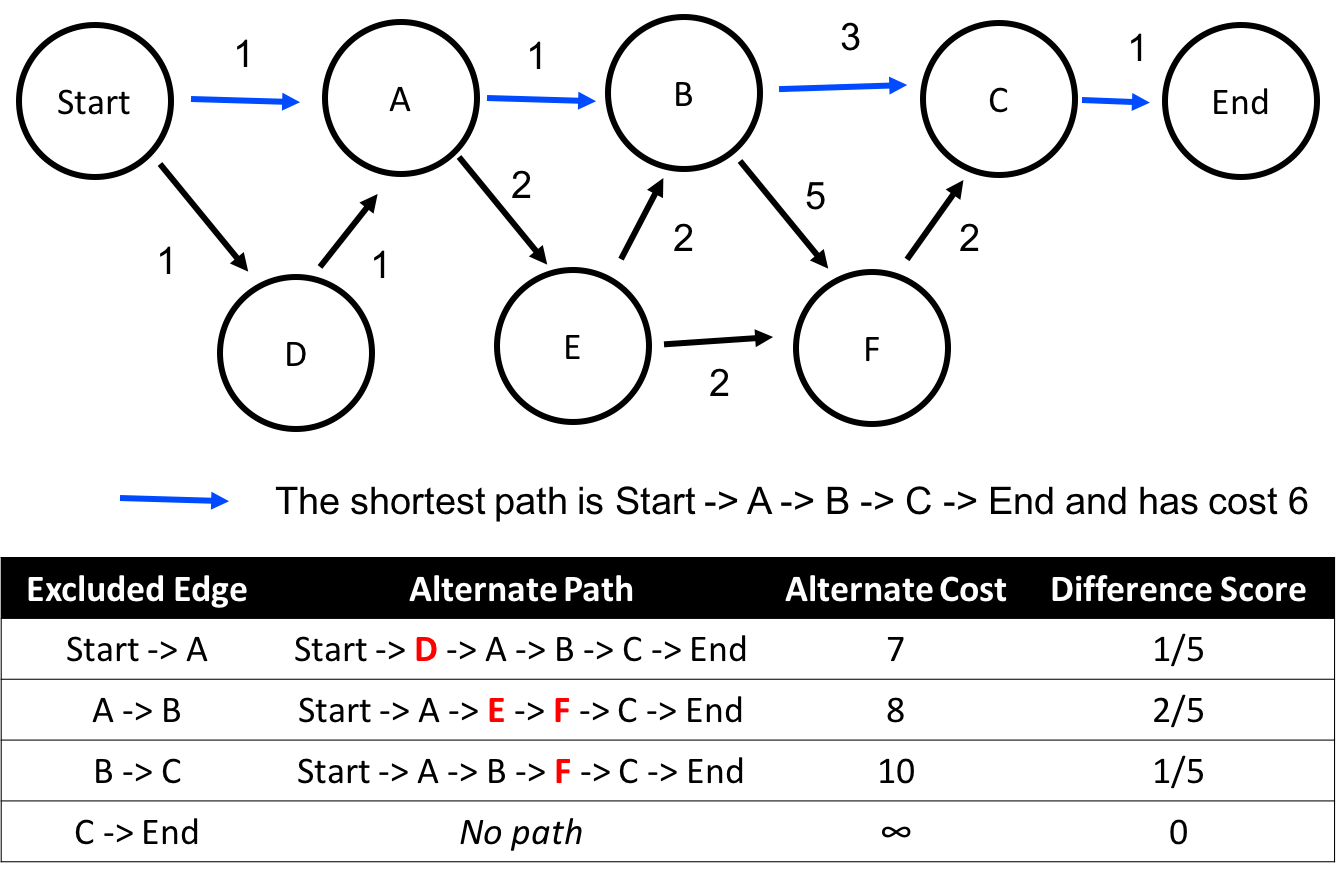The shortest alternate route is the path Start -> D -> A -> B -> C -> End. However it has a difference score of 0.2 (recall that we are looking for a path with difference score greater than 0.2). The shortest alternative that also has a difference greater that 0.2 is:
    Start -> A -> E -> F -> C -> End
That is the path that your alternative path algorithm returns.

There are different ways to accomplish the modification to your path algorithm. One option is to simply write a separate and modified Dijkstra or A* function that has the ability to ignore an edge. While this option is allowed for your project, it does duplicate a lot of code. A better option would be to refactor your code and write a modified function that performs Dijstra or A* and can either perform the standard algorithm or can ignore a given edge. Then, modify your original Dijkstra or A* function to simply call your new function.

## Creative Aspect

Turn in files map-custom.txt and map-custom.jpg representing a map graph of your own. Put the files into the res/ folder of your project. The text file contains information about the graph's vertexes and edges. The graph can be whatever you want, so long as it is not essentially the same as any of the provided graphs. Your image can be any (non-offensive) JPEG image you like; we encourage you to use a search engine like Google Image Search to find an interesting background. The text file's format should exactly match the following example, from map-small.txt. For full credit, your file should load successfully into the program without causing an error and be searchable by the user.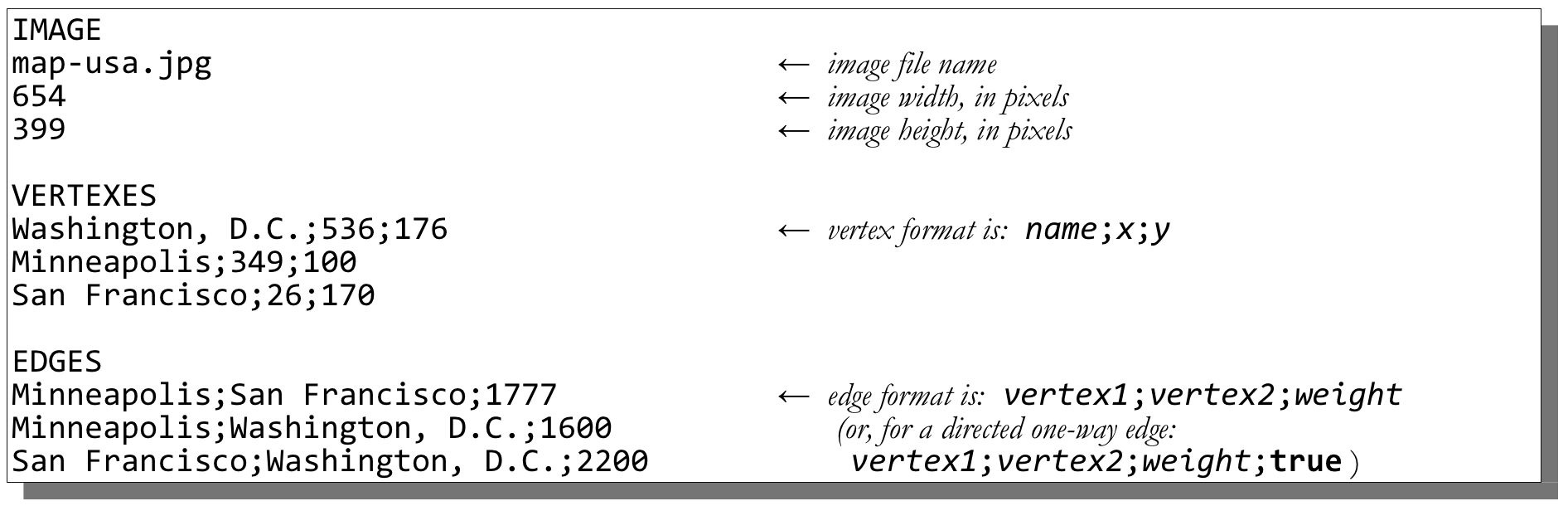## Development Strategy

• Trace through the algorithms by hand on small sample graphs before coding them.
• Work step-by-step. Complete each algorithm before starting the next one. You can test each individually even if others are incomplete. We suggest doing BFS, then Dijkstra's, then A*, and finally Alternative Route.
• Start out with tiny worlds first. It is much easier to trace your algorithm and/or print every step of its execution if the world is small. Try small or middle earth before trying one of the large graphs (especially Stanford, Istanbul or San Francisco)
• Remember that edge costs are doubles, not ints.
• In both A* and Dijkstra you will need to calculate the cost of a path. You can (but don't have to) memoize the cost calculation.
• In Dijkstra's algorithm, don't stop your algorithm early when you enqueue the ending vertex; stop it when you dequeue the ending vertex (that is, when you color the vertex green).
• The alternate path algorithm that we ask you to implement is slow. Test it on small maps. On the Stanford map it can take seconds and on Istanbul and San Francisco it can take minutes.